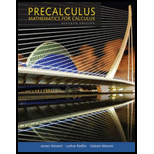Chapter 5.3, Problem 67E### Precalculus: Mathematics for Calcu...

7th Edition
James Stewart + 2 others
ISBN: 9781305071759

#### Solutions

Chapter
Section### Precalculus: Mathematics for Calcu...

7th Edition
James Stewart + 2 others
ISBN: 9781305071759
Textbook Problem

# Sine and Cosine Curves with Variable Amplitude Graph the three functions on a common screen. How are the graphs related?67. y = x2, y = −x2, y = x2 sin x

To determine

To sketch: The graph of the functions and obtain the relation.

Explanation

Given:

The functions are y=x2,y=x2 and y=x2sinx .

Calculation:

The range of the sine function is 1sinx1 .

Multiply the above inequalities by x2 ,

x2x2sinxx2

Thus, the value of y=x2sinx is lies between the functions y=x2 and y=x2

### Still sussing out bartleby?

Check out a sample textbook solution.

See a sample solution

#### The Solution to Your Study Problems

Bartleby provides explanations to thousands of textbook problems written by our experts, many with advanced degrees!

Get Started

## Additional Math Solutions

#### Expand each expression in Exercises 122. (y1y)(y+1y)

Finite Mathematics and Applied Calculus (MindTap Course List)

#### Differentiate. y=x2tanx

Calculus: Early Transcendentals

#### Multiply: 70601300

Elementary Technical Mathematics

#### Multiply. (3sin+4)(5cos+2)

Trigonometry (MindTap Course List)

#### For f(x) = x3 + 7x, df = _____. a) (x3 + 7x) dx b) (3x2 + 7) dx c) 3x2 + 7 d) x3 + 7x + dx

Study Guide for Stewart's Single Variable Calculus: Early Transcendentals, 8th